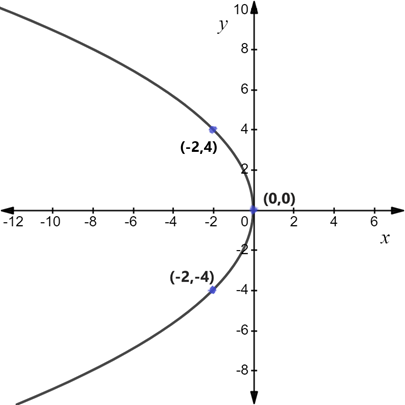# To graph: The equation 8 x + y 2 = 0 and check the equation for symmetry.### Precalculus: Mathematics for Calcu...

6th Edition
Stewart + 5 others
Publisher: Cengage Learning
ISBN: 9780840068071### Precalculus: Mathematics for Calcu...

6th Edition
Stewart + 5 others
Publisher: Cengage Learning
ISBN: 9780840068071

#### Solutions

Chapter 1, Problem 116RE
To determine

## To graph: The equation 8x+y2=0 and check the equation for symmetry.

Expert Solution

The equation 8x+y2=0 is symmetric about x -axis but not about y -axis and the graph is as follows:### Explanation of Solution

Given information:

The equation of line 8x+y2=0

Formula used:

The equation is said to have symmetry about the x -axis when the equation remains unchanged on replacing y by y

Graphically it can be said that the graph remains unchanged when reflected in the x -axis.

The equation is said to have symmetry about the y -axis when the equation remains unchanged on replacing x by x

Graphically it can be said that the graph remains unchanged when reflected in the y -axis.

The equation is said to have symmetry about the origin if it is symmetric about x -axis and y -axis that is the equation remains unchanged on replacing x by x or y by y

Graphically it can be said that the graph remains unchanged when rotated with an angle of 180 about the origin.

Calculation:

It is provided that the equation of line is 8x+y2=0 (1)

Firstly replace y by y in (1)

8x+(y)2=08x+y2=0

The equation obtained is the same as the original one.

Hence, 8x+y2=0 is symmetric about x -axis

Next, replace x by x in (1)

8(x)+y2=08x+y2=0

The equation obtained isnot the same as the original one.

Hence, 8x+y2=0 isnot symmetric about y -axis.

For plotting:

 x y=16−x2 (x.y) 0−2−2 04−4 (0,0)(−2,4)(−2,−4)

We plot these points to get the graph as follows: